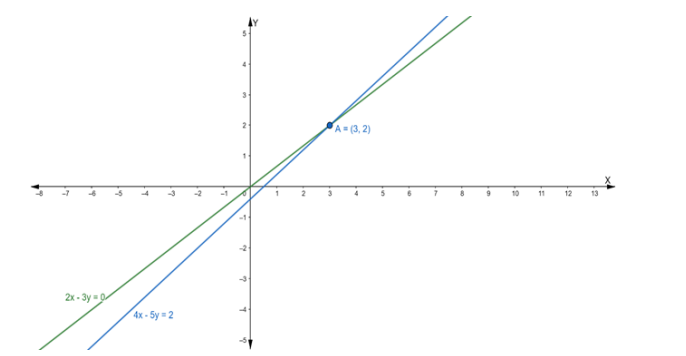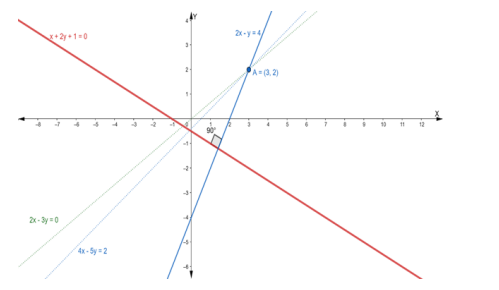# Find the equation of the line through the intersection of the lines`
Question:

Find the equation of the line through the intersection of the lines 2x – 3y = 0 and $4 x-5 y=2$ and which is perpendicular to the line $x+2 y+1=0$

Solution:

Suppose the given two lines intersect at a point $P\left(x_{1}, y_{1}\right) .$ Then, $\left(x_{1}, y_{1}\right)$ satisfies each of the given equations.

$2 x-3 y=0 \ldots$ (i)

$4 x-5 y=2 \ldots$ (ii)

Now, we find the point of intersection of eq. (i) and (ii)

Multiply the eq. (i) by 2, we get

$4 x-6 y=0 \ldots$ (iii)

On subtracting eq. (iii) from (ii), we get

$4 x-5 y-4 x+6 y=2-0$

$\Rightarrow y=2$

Putting the value of y in eq. (i), we get

$2 x-3(2)=0$

$\Rightarrow 2 x-6=0$

$\Rightarrow 2 x=6$

$\Rightarrow x=3$

Hence, the point of intersection $P\left(x_{1}, y_{1}\right)$ is $(3,2)$Now, we know that, when two lines are perpendicular, then the product of their slope is equal to $-1$

$m_{1} \times m_{2}=-1$

$\Rightarrow$ Slope of the given line $\times$ Slope of the perpendicular line $=-1$

$\therefore\left(-\frac{1}{2}\right) \times$ Slope of the perpendicular line $=-1$

$\Rightarrow$ The slope of the perpendicular line $=2$

So, the slope of a line which is perpendicular to the given line is 2

Then the equation of the line passing through the point $(3,2)$ having slope 2 is:

$y-y_{1}=m\left(x-x_{1}\right)$

$\Rightarrow y-2=2(x-3)$

$\Rightarrow y-2=2 x-6$

$\Rightarrow 2 x-y-6+2=0$

$\Rightarrow 2 x-y-4=0$